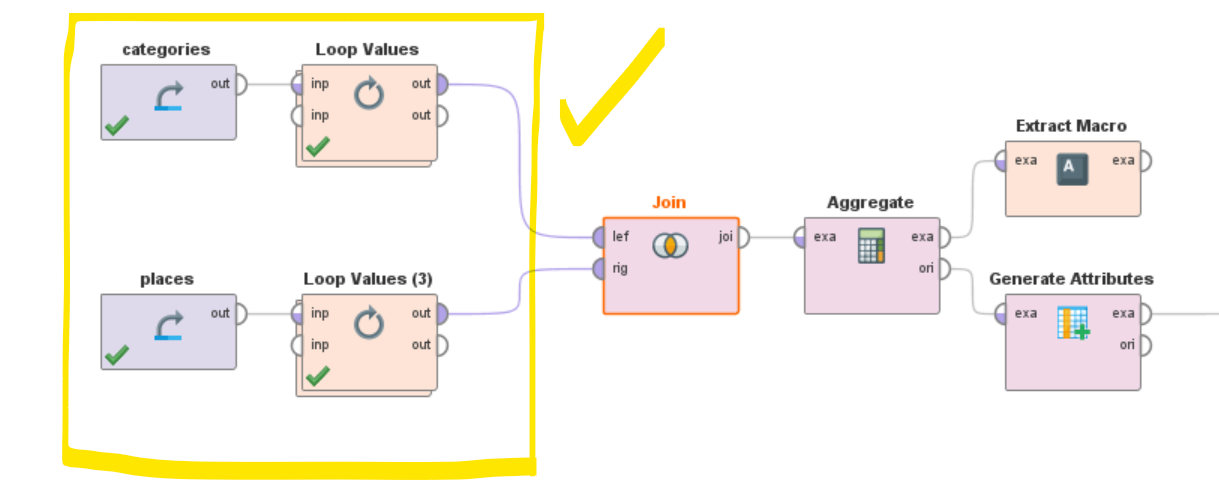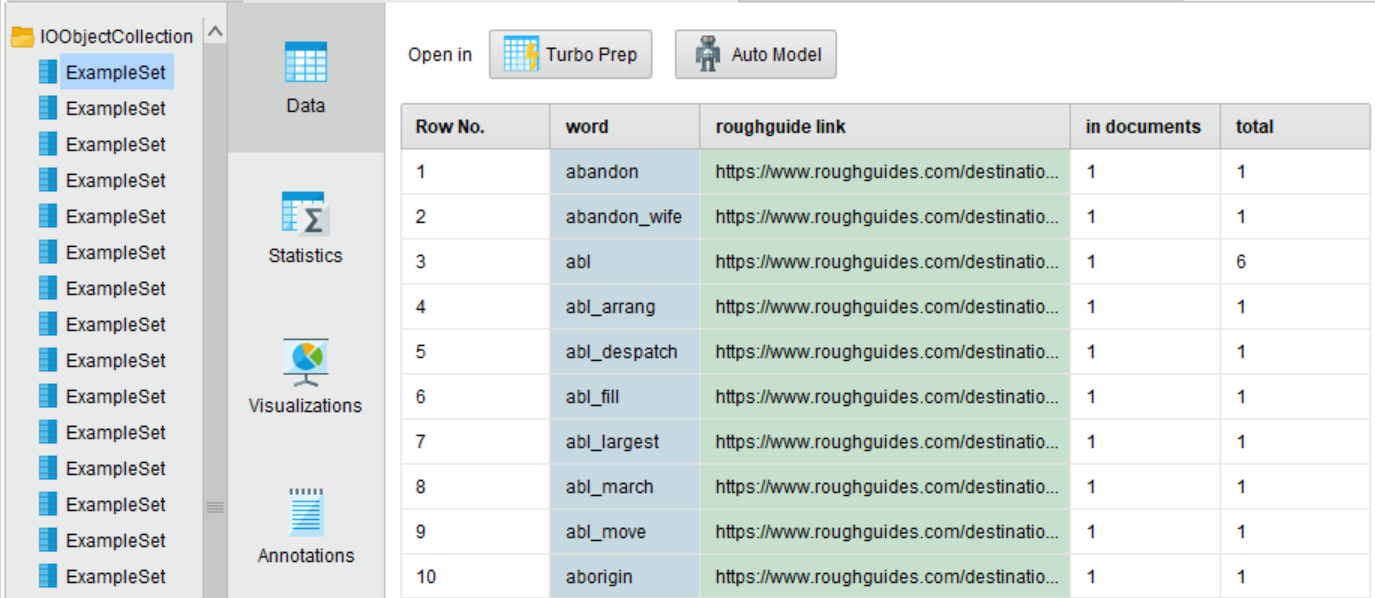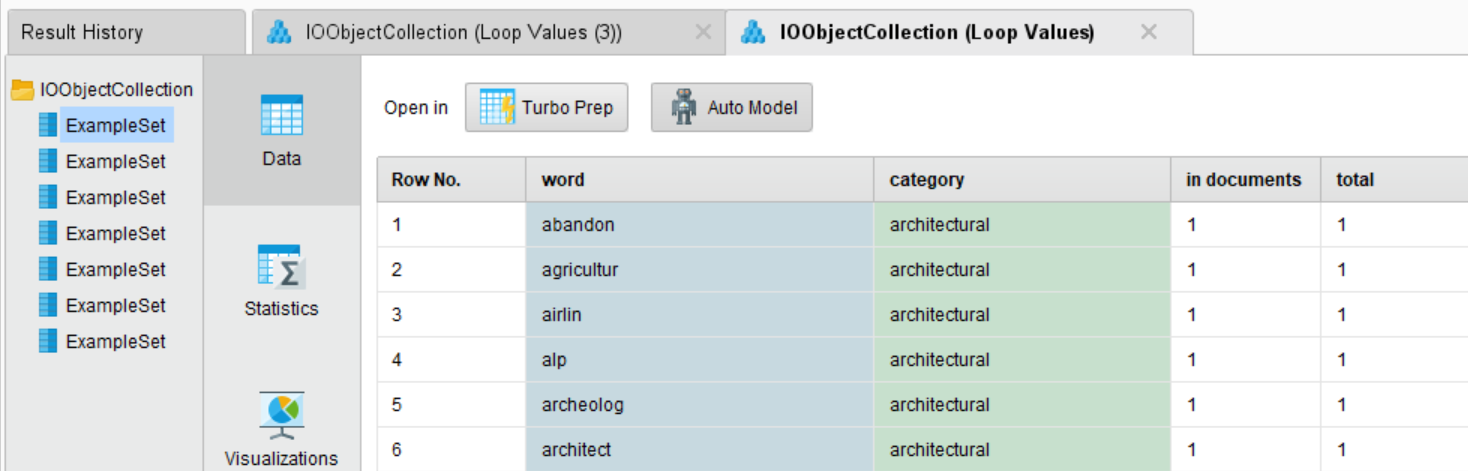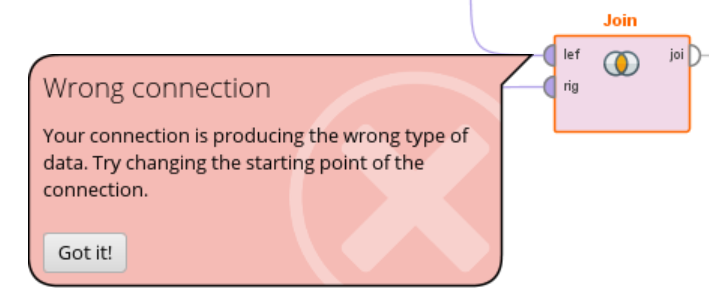# Automate manual iterations // Text analysis

Member Posts: 21Contributor II
Hello Community,

I am facing an automation problem.

Initial situation:
• I want to check how often words from a given list (categories) occur in my Example Sets (100 texts).
• I have 7 categories with labelled words (architecture, activities, culinary etc.)
• So the point is to check in 100 texts how many words occur per category.

Example (Culinary category):
• In the Culinary list there are words like "curry", "spaghetti" and "pizza".
• In the first text there are e.g. 2 hits (curry + pizza). So the final result for text 1 will be 2 for the category "Culinary".
• Next follows the 2nd category "Architecture" and so on.
• When all categories have been passed through, the second text is considered.
At the end we have a result, which words from the categories occur how often in the individual texts. From this we can then conclude what weighting the category has for the text.

The process is already running but only manually. So I have to change the parameters manually 100 x 7 times (Filter Example Range), which is not very nice. Is there a way how I can automatically run the lists against each other?

Routine (idea):
1. take category 1 and check in which texts from 1-100 the words occur how often.
2. take category 2 and check in which texts from 1-100 the words occur how often.

I hope you understand my problem and can help me! I have attached all relevant data.

Best regards,

Patrick

• Administrator, Employee, RapidMiner Certified Analyst, Member Posts: 271Unicorn
@Hyperrick I need to go through your data but it seems you could solve it with a loop values operator since you said that the basic process is working for you.

You'll need a Data Set that could look something like
Category ----|---- Word
Architecture   Word1
Architecture   Word2
Architecture   .......n
Food               Word1
Food               Word2
Food               ......n

With the loop values you will pick Category as the attribute you are going to loop and in the inner process you could filter examples that contain the category that the macro took on each iteration and then run your process.

Hope this helps.

• Member Posts: 21Contributor II
Hi Marco,

I prepared the data as you said and am now able to have both lists as collections prepared.

Process overview (first part [yellow higlighted] works with your solution):Result1: Collection of categories with words; ID is "word", label is "url":Result 2: Collection of texts with words; ID is "word", label is "url":The next step is to join only matching words together with the operator inner join. Unfortunately I have no idea to build the process from now on. The join doesn't work showing following error message:Do I have to build another Loop operator around the join (and following oeprators "aggregate" etc.)?

```<?xml version="1.0" encoding="UTF-8"?><process version="9.8.000">
<context>
<input/>
<output/>
<macros/>
</context>
<operator activated="true" class="process" compatibility="9.8.000" expanded="true" name="Process">
<parameter key="logverbosity" value="init"/>
<parameter key="random_seed" value="2001"/>
<parameter key="send_mail" value="never"/>
<parameter key="process_duration_for_mail" value="30"/>
<parameter key="encoding" value="SYSTEM"/>
<process expanded="true">
<operator activated="true" class="retrieve" compatibility="9.8.000" expanded="true" height="68" name="categories" width="90" x="179" y="34">
<parameter key="repository_entry" value="../data/categories_and_words"/>
</operator>
<operator activated="true" class="concurrency:loop_values" compatibility="9.8.000" expanded="true" height="82" name="Loop Values" width="90" x="313" y="34">
<parameter key="attribute" value="category"/>
<parameter key="iteration_macro" value="loop_value"/>
<parameter key="reuse_results" value="false"/>
<parameter key="enable_parallel_execution" value="true"/>
<process expanded="true">
<operator activated="true" class="filter_examples" compatibility="9.8.000" expanded="true" height="103" name="Filter Examples" width="90" x="112" y="34">
<parameter key="parameter_string" value="category = %{loop_value}"/>
<parameter key="parameter_expression" value=""/>
<parameter key="condition_class" value="attribute_value_filter"/>
<parameter key="invert_filter" value="false"/>
<list key="filters_list"/>
<parameter key="filters_logic_and" value="true"/>
</operator>
<operator activated="true" class="extract_macro" compatibility="9.8.000" expanded="true" height="68" name="Extract Macro (3)" width="90" x="246" y="34">
<parameter key="macro" value="category"/>
<parameter key="macro_type" value="data_value"/>
<parameter key="statistics" value="average"/>
<parameter key="attribute_name" value="category"/>
<parameter key="example_index" value="1"/>
</operator>
<operator activated="true" class="nominal_to_text" compatibility="9.8.000" expanded="true" height="82" name="Nominal to Text" width="90" x="380" y="34">
<parameter key="attribute_filter_type" value="all"/>
<parameter key="attribute" value=""/>
<parameter key="attributes" value=""/>
<parameter key="use_except_expression" value="false"/>
<parameter key="value_type" value="nominal"/>
<parameter key="use_value_type_exception" value="false"/>
<parameter key="except_value_type" value="file_path"/>
<parameter key="block_type" value="single_value"/>
<parameter key="use_block_type_exception" value="false"/>
<parameter key="except_block_type" value="single_value"/>
<parameter key="invert_selection" value="false"/>
<parameter key="include_special_attributes" value="false"/>
</operator>
<operator activated="true" class="text:process_document_from_data" compatibility="9.3.001" expanded="true" height="82" name="Process Documents from Data" width="90" x="514" y="34">
<parameter key="create_word_vector" value="true"/>
<parameter key="vector_creation" value="Binary Term Occurrences"/>
<parameter key="keep_text" value="false"/>
<parameter key="prune_method" value="none"/>
<parameter key="prune_below_percent" value="3.0"/>
<parameter key="prune_above_percent" value="30.0"/>
<parameter key="prune_below_rank" value="0.05"/>
<parameter key="prune_above_rank" value="0.95"/>
<parameter key="datamanagement" value="double_sparse_array"/>
<parameter key="data_management" value="auto"/>
<parameter key="select_attributes_and_weights" value="false"/>
<list key="specify_weights"/>
<process expanded="true">
<operator activated="true" class="text:tokenize" compatibility="9.3.001" expanded="true" height="68" name="Tokenize" width="90" x="112" y="34">
<parameter key="mode" value="non letters"/>
<parameter key="characters" value=".:"/>
<parameter key="language" value="English"/>
<parameter key="max_token_length" value="3"/>
</operator>
<operator activated="true" class="text:stem_porter" compatibility="9.3.001" expanded="true" height="68" name="Stem (Porter)" width="90" x="246" y="34"/>
<operator activated="true" class="text:generate_n_grams_terms" compatibility="9.3.001" expanded="true" height="68" name="Generate n-Grams (Terms)" width="90" x="380" y="34">
<parameter key="max_length" value="2"/>
</operator>
<operator activated="true" class="text:filter_stopwords_english" compatibility="9.3.001" expanded="true" height="68" name="Filter Stopwords (English)" width="90" x="514" y="34"/>
<connect from_port="document" to_op="Tokenize" to_port="document"/>
<connect from_op="Tokenize" from_port="document" to_op="Stem (Porter)" to_port="document"/>
<connect from_op="Stem (Porter)" from_port="document" to_op="Generate n-Grams (Terms)" to_port="document"/>
<connect from_op="Generate n-Grams (Terms)" from_port="document" to_op="Filter Stopwords (English)" to_port="document"/>
<connect from_op="Filter Stopwords (English)" from_port="document" to_port="document 1"/>
<portSpacing port="source_document" spacing="0"/>
<portSpacing port="sink_document 1" spacing="0"/>
<portSpacing port="sink_document 2" spacing="0"/>
</process>
</operator>
<operator activated="true" class="text:wordlist_to_data" compatibility="9.3.001" expanded="true" height="82" name="WordList to Data" width="90" x="648" y="34"/>
<operator activated="true" class="generate_attributes" compatibility="9.8.000" expanded="true" height="82" name="Generate Attributes (3)" width="90" x="782" y="34">
<list key="function_descriptions">
<parameter key="category" value="%{category}"/>
</list>
<parameter key="keep_all" value="true"/>
</operator>
<operator activated="true" class="set_role" compatibility="9.8.000" expanded="true" height="82" name="Set Role (2)" width="90" x="916" y="34">
<parameter key="attribute_name" value="category"/>
<parameter key="target_role" value="label"/>
<parameter key="word" value="id"/>
</list>
</operator>
<operator activated="true" class="remove_attribute_range" compatibility="9.8.000" expanded="true" height="82" name="Remove Attribute Range" width="90" x="1050" y="34">
<parameter key="first_attribute" value="4"/>
<parameter key="last_attribute" value="9"/>
</operator>
<operator activated="true" class="remove_attribute_range" compatibility="9.8.000" expanded="true" height="82" name="Remove Attribute Range (3)" width="90" x="1184" y="34">
<parameter key="first_attribute" value="3"/>
<parameter key="last_attribute" value="3"/>
</operator>
<connect from_port="input 1" to_op="Filter Examples" to_port="example set input"/>
<connect from_op="Filter Examples" from_port="example set output" to_op="Extract Macro (3)" to_port="example set"/>
<connect from_op="Extract Macro (3)" from_port="example set" to_op="Nominal to Text" to_port="example set input"/>
<connect from_op="Nominal to Text" from_port="example set output" to_op="Process Documents from Data" to_port="example set"/>
<connect from_op="Process Documents from Data" from_port="word list" to_op="WordList to Data" to_port="word list"/>
<connect from_op="WordList to Data" from_port="example set" to_op="Generate Attributes (3)" to_port="example set input"/>
<connect from_op="Generate Attributes (3)" from_port="example set output" to_op="Set Role (2)" to_port="example set input"/>
<connect from_op="Set Role (2)" from_port="example set output" to_op="Remove Attribute Range" to_port="example set input"/>
<connect from_op="Remove Attribute Range" from_port="example set output" to_op="Remove Attribute Range (3)" to_port="example set input"/>
<connect from_op="Remove Attribute Range (3)" from_port="example set output" to_port="output 1"/>
<portSpacing port="source_input 1" spacing="0"/>
<portSpacing port="source_input 2" spacing="0"/>
<portSpacing port="sink_output 1" spacing="0"/>
<portSpacing port="sink_output 2" spacing="0"/>
</process>
</operator>
<operator activated="true" class="retrieve" compatibility="9.8.000" expanded="true" height="68" name="places" width="90" x="179" y="187">
<parameter key="repository_entry" value="../data/prepared_data_asia"/>
</operator>
<operator activated="true" class="concurrency:loop_values" compatibility="9.8.000" expanded="true" height="82" name="Loop Values (3)" width="90" x="313" y="187">
<parameter key="iteration_macro" value="loop_value"/>
<parameter key="reuse_results" value="false"/>
<parameter key="enable_parallel_execution" value="true"/>
<process expanded="true">
<operator activated="true" class="filter_examples" compatibility="9.8.000" expanded="true" height="103" name="Filter Examples (3)" width="90" x="112" y="34">
<parameter key="parameter_string" value="roughguide link = %{loop_value}"/>
<parameter key="parameter_expression" value=""/>
<parameter key="condition_class" value="attribute_value_filter"/>
<parameter key="invert_filter" value="false"/>
<list key="filters_list"/>
<parameter key="filters_logic_and" value="true"/>
</operator>
<operator activated="true" class="extract_macro" compatibility="9.8.000" expanded="true" height="68" name="Extract Macro (2)" width="90" x="246" y="34">
<parameter key="macro" value="place"/>
<parameter key="macro_type" value="data_value"/>
<parameter key="statistics" value="average"/>
<parameter key="example_index" value="1"/>
</operator>
<operator activated="true" class="nominal_to_text" compatibility="9.8.000" expanded="true" height="82" name="Nominal to Text (3)" width="90" x="380" y="34">
<parameter key="attribute_filter_type" value="all"/>
<parameter key="attribute" value=""/>
<parameter key="attributes" value=""/>
<parameter key="use_except_expression" value="false"/>
<parameter key="value_type" value="nominal"/>
<parameter key="use_value_type_exception" value="false"/>
<parameter key="except_value_type" value="file_path"/>
<parameter key="block_type" value="single_value"/>
<parameter key="use_block_type_exception" value="false"/>
<parameter key="except_block_type" value="single_value"/>
<parameter key="invert_selection" value="false"/>
<parameter key="include_special_attributes" value="false"/>
</operator>
<operator activated="true" class="text:process_document_from_data" compatibility="9.3.001" expanded="true" height="82" name="Process Documents from Data (3)" width="90" x="514" y="34">
<parameter key="create_word_vector" value="true"/>
<parameter key="vector_creation" value="Term Occurrences"/>
<parameter key="keep_text" value="false"/>
<parameter key="prune_method" value="none"/>
<parameter key="prune_below_percent" value="3.0"/>
<parameter key="prune_above_percent" value="30.0"/>
<parameter key="prune_below_rank" value="0.05"/>
<parameter key="prune_above_rank" value="0.95"/>
<parameter key="datamanagement" value="double_sparse_array"/>
<parameter key="data_management" value="auto"/>
<parameter key="select_attributes_and_weights" value="false"/>
<list key="specify_weights"/>
<process expanded="true">
<operator activated="true" class="text:tokenize" compatibility="9.3.001" expanded="true" height="68" name="Tokenize (4)" width="90" x="112" y="34">
<parameter key="mode" value="non letters"/>
<parameter key="characters" value=".:"/>
<parameter key="language" value="English"/>
<parameter key="max_token_length" value="3"/>
</operator>
<operator activated="true" class="text:stem_porter" compatibility="9.3.001" expanded="true" height="68" name="Stem (Porter) (4)" width="90" x="246" y="34"/>
<operator activated="true" class="text:generate_n_grams_terms" compatibility="9.3.001" expanded="true" height="68" name="Generate n-Grams (Terms) (3)" width="90" x="380" y="34">
<parameter key="max_length" value="2"/>
</operator>
<operator activated="true" class="text:filter_stopwords_english" compatibility="9.3.001" expanded="true" height="68" name="Filter Stopwords (English) (4)" width="90" x="514" y="34"/>
<connect from_port="document" to_op="Tokenize (4)" to_port="document"/>
<connect from_op="Tokenize (4)" from_port="document" to_op="Stem (Porter) (4)" to_port="document"/>
<connect from_op="Stem (Porter) (4)" from_port="document" to_op="Generate n-Grams (Terms) (3)" to_port="document"/>
<connect from_op="Generate n-Grams (Terms) (3)" from_port="document" to_op="Filter Stopwords (English) (4)" to_port="document"/>
<connect from_op="Filter Stopwords (English) (4)" from_port="document" to_port="document 1"/>
<portSpacing port="source_document" spacing="0"/>
<portSpacing port="sink_document 1" spacing="0"/>
<portSpacing port="sink_document 2" spacing="0"/>
</process>
</operator>
<operator activated="true" class="text:wordlist_to_data" compatibility="9.3.001" expanded="true" height="82" name="WordList to Data (4)" width="90" x="648" y="34"/>
<operator activated="true" class="generate_attributes" compatibility="9.8.000" expanded="true" height="82" name="Generate Attributes (2)" width="90" x="782" y="34">
<list key="function_descriptions">
</list>
<parameter key="keep_all" value="true"/>
</operator>
<operator activated="true" class="set_role" compatibility="9.8.000" expanded="true" height="82" name="Set Role" width="90" x="916" y="34">
<parameter key="target_role" value="label"/>
<parameter key="word" value="id"/>
</list>
</operator>
<connect from_port="input 1" to_op="Filter Examples (3)" to_port="example set input"/>
<connect from_op="Filter Examples (3)" from_port="example set output" to_op="Extract Macro (2)" to_port="example set"/>
<connect from_op="Extract Macro (2)" from_port="example set" to_op="Nominal to Text (3)" to_port="example set input"/>
<connect from_op="Nominal to Text (3)" from_port="example set output" to_op="Process Documents from Data (3)" to_port="example set"/>
<connect from_op="Process Documents from Data (3)" from_port="word list" to_op="WordList to Data (4)" to_port="word list"/>
<connect from_op="WordList to Data (4)" from_port="example set" to_op="Generate Attributes (2)" to_port="example set input"/>
<connect from_op="Generate Attributes (2)" from_port="example set output" to_op="Set Role" to_port="example set input"/>
<connect from_op="Set Role" from_port="example set output" to_port="output 1"/>
<portSpacing port="source_input 1" spacing="0"/>
<portSpacing port="source_input 2" spacing="0"/>
<portSpacing port="sink_output 1" spacing="0"/>
<portSpacing port="sink_output 2" spacing="0"/>
</process>
</operator>
<operator activated="true" class="concurrency:join" compatibility="9.8.000" expanded="true" height="82" name="Join" width="90" x="514" y="136">
<parameter key="remove_double_attributes" value="true"/>
<parameter key="join_type" value="inner"/>
<parameter key="use_id_attribute_as_key" value="true"/>
<list key="key_attributes">
<parameter key="word" value="word"/>
</list>
<parameter key="keep_both_join_attributes" value="false"/>
</operator>
<operator activated="true" class="aggregate" compatibility="7.4.000" expanded="true" height="82" name="Aggregate" width="90" x="648" y="136">
<parameter key="use_default_aggregation" value="false"/>
<parameter key="attribute_filter_type" value="all"/>
<parameter key="attribute" value=""/>
<parameter key="attributes" value=""/>
<parameter key="use_except_expression" value="false"/>
<parameter key="value_type" value="attribute_value"/>
<parameter key="use_value_type_exception" value="false"/>
<parameter key="except_value_type" value="time"/>
<parameter key="block_type" value="attribute_block"/>
<parameter key="use_block_type_exception" value="false"/>
<parameter key="except_block_type" value="value_matrix_row_start"/>
<parameter key="invert_selection" value="false"/>
<parameter key="include_special_attributes" value="false"/>
<parameter key="default_aggregation_function" value="average"/>
<list key="aggregation_attributes">
<parameter key="total" value="sum"/>
</list>
<parameter key="group_by_attributes" value=""/>
<parameter key="count_all_combinations" value="false"/>
<parameter key="only_distinct" value="false"/>
<parameter key="ignore_missings" value="true"/>
</operator>
<operator activated="true" class="extract_macro" compatibility="9.8.000" expanded="true" height="68" name="Extract Macro" width="90" x="782" y="85">
<parameter key="macro" value="matches"/>
<parameter key="macro_type" value="data_value"/>
<parameter key="statistics" value="average"/>
<parameter key="attribute_name" value="sum(total)"/>
<parameter key="example_index" value="1"/>
</operator>
<operator activated="true" class="generate_attributes" compatibility="9.8.000" expanded="true" height="82" name="Generate Attributes" width="90" x="782" y="187">
<list key="function_descriptions">
<parameter key="matches" value="%{matches}"/>
<parameter key="category" value="%{category}"/>
</list>
<parameter key="keep_all" value="true"/>
</operator>
<connect from_op="categories" from_port="output" to_op="Loop Values" to_port="input 1"/>
<connect from_op="Loop Values" from_port="output 1" to_op="Join" to_port="left"/>
<connect from_op="places" from_port="output" to_op="Loop Values (3)" to_port="input 1"/>
<connect from_op="Loop Values (3)" from_port="output 1" to_op="Join" to_port="right"/>
<connect from_op="Join" from_port="join" to_op="Aggregate" to_port="example set input"/>
<connect from_op="Aggregate" from_port="example set output" to_op="Extract Macro" to_port="example set"/>
<connect from_op="Aggregate" from_port="original" to_op="Generate Attributes" to_port="example set input"/>
<connect from_op="Generate Attributes" from_port="example set output" to_port="result 1"/>
<portSpacing port="source_input 1" spacing="0"/>
<portSpacing port="sink_result 1" spacing="0"/>
<portSpacing port="sink_result 2" spacing="0"/>
</process>
</operator>
</process>
```

Kind regards,

Patrick
• Member Posts: 21Contributor II
Hi Marco,

thanks for your answer. Great, you helped me out and I learned a lot!

Kind regards,

Patrick